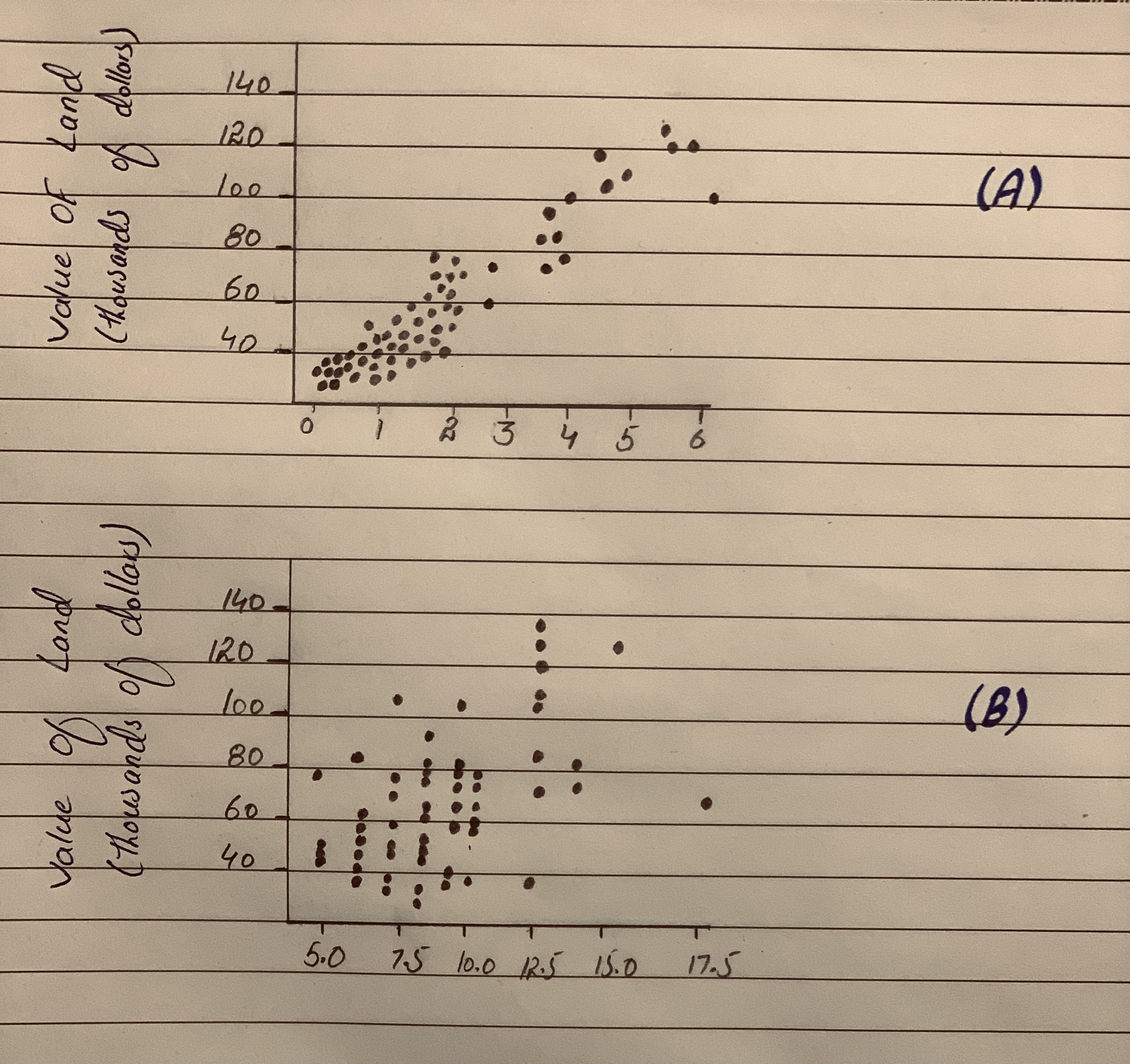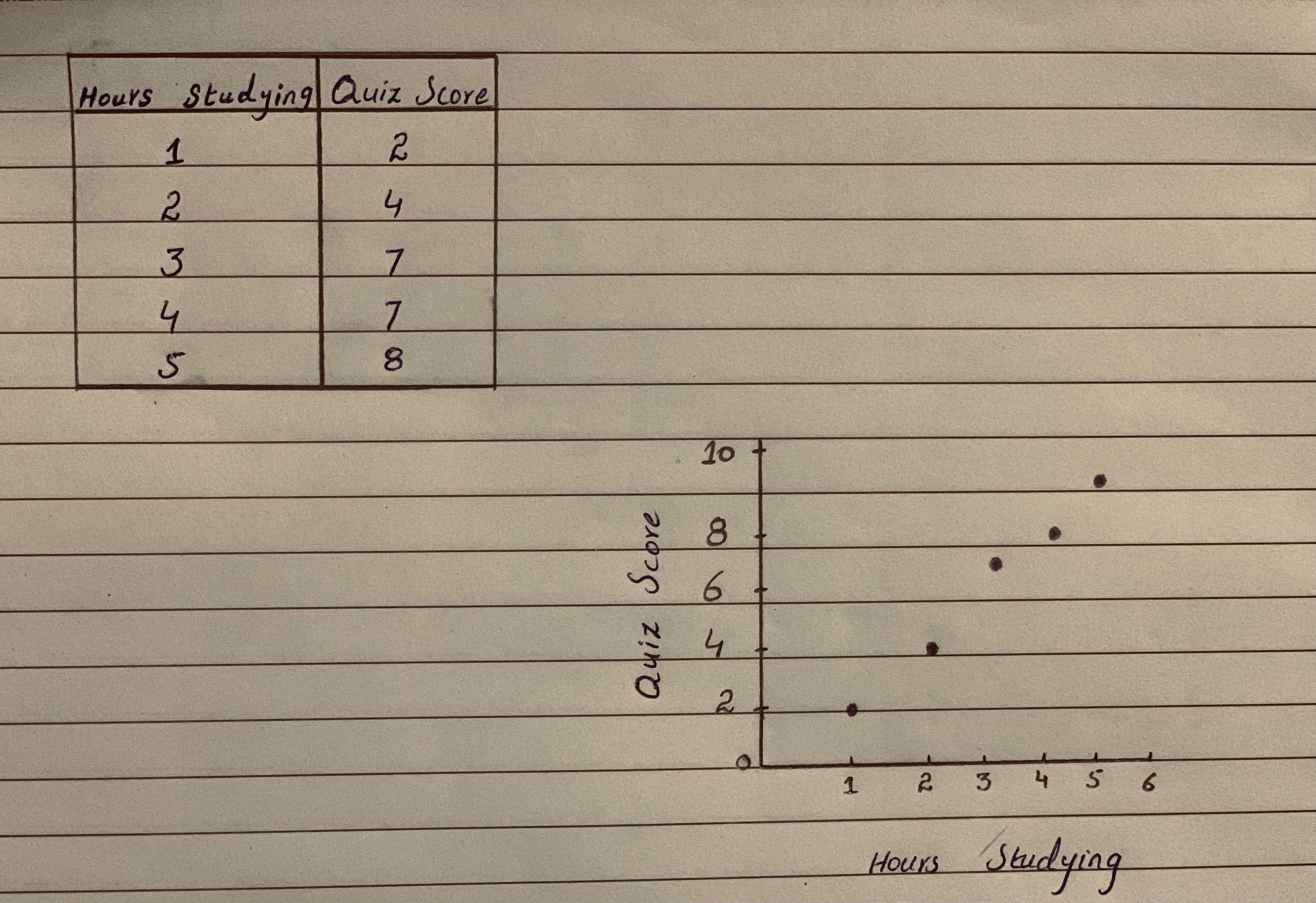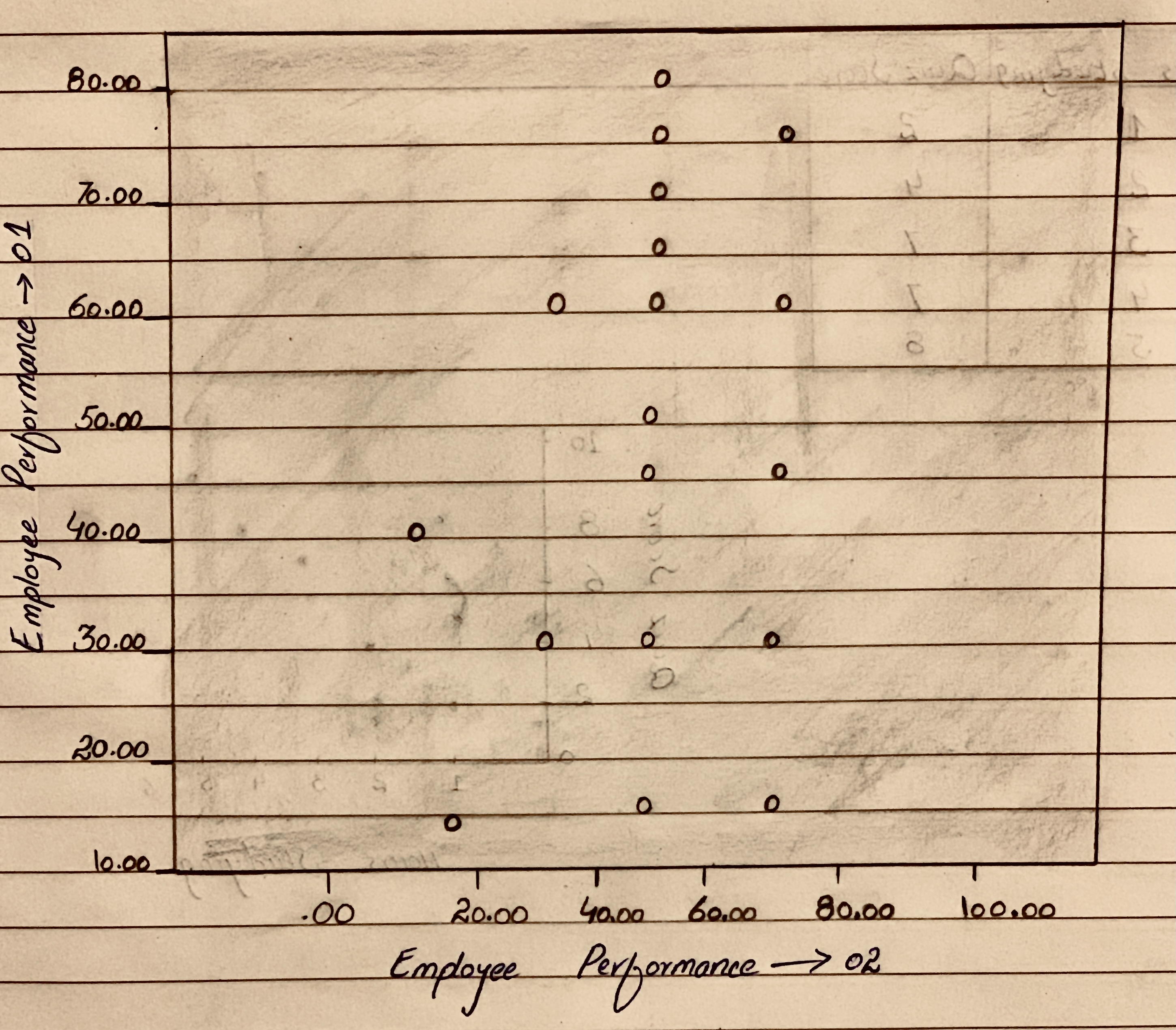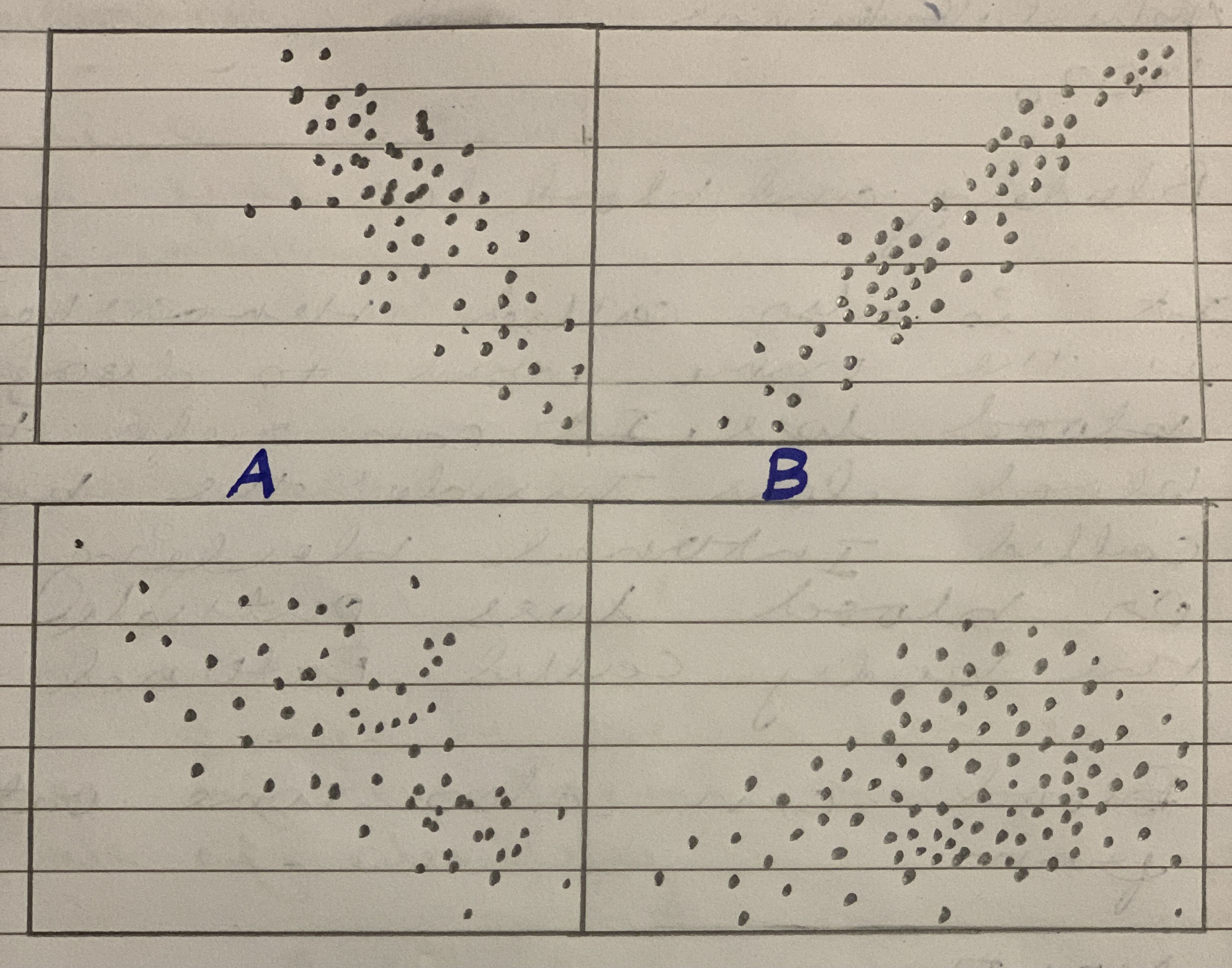# Give relations mentioned in the given scatterplots A & BQuestion
ScatterplotsGive relations mentioned in the given scatterplots A & B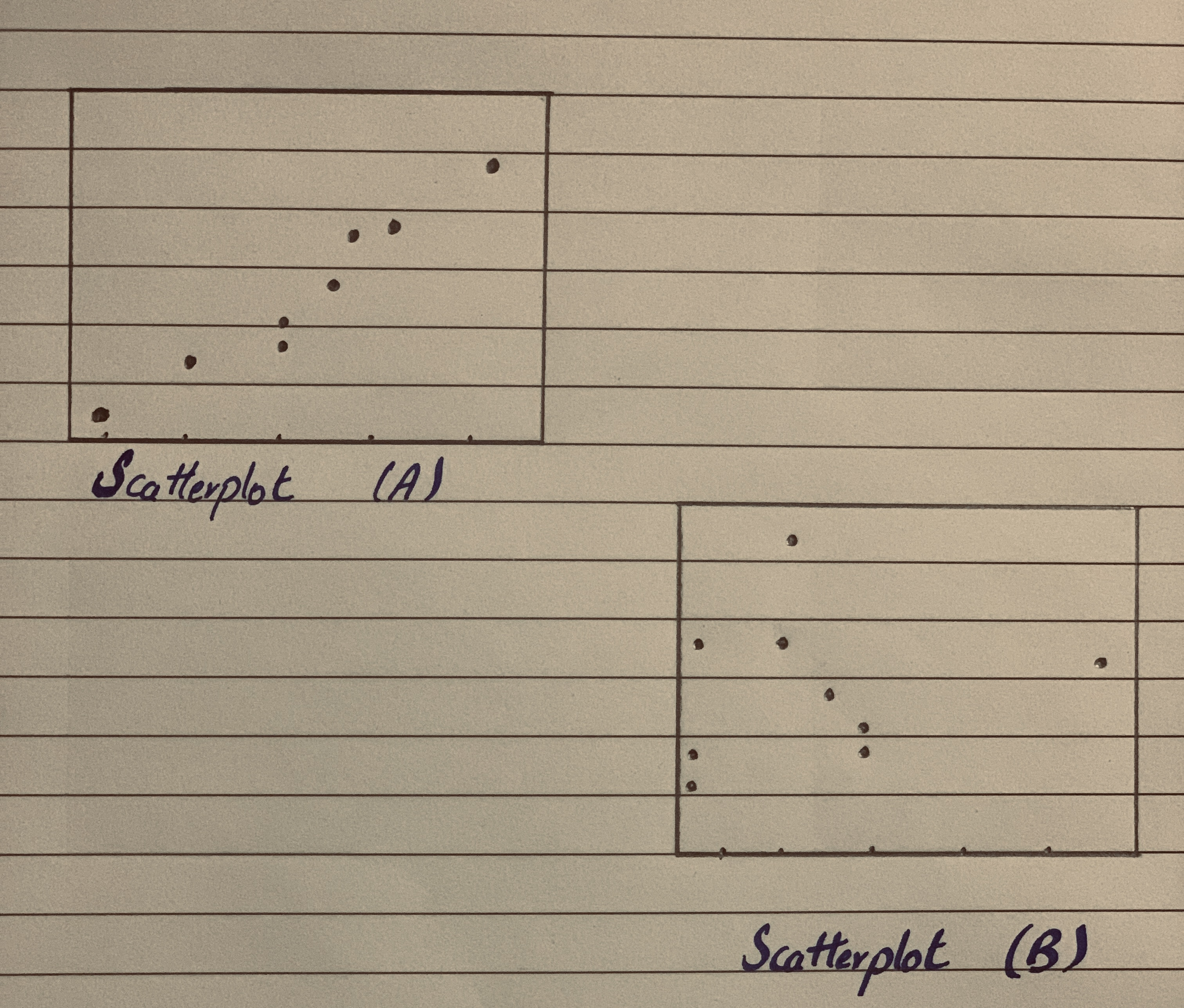2021-02-27
Relationship between two variables:
Scatterplots A:
In this scatter plot, it is clear that both variables are in same direction. That is, both quantitative variables are increasing or decreasing together and it is approximately linear. Therefore, the relationship is positive linear.
Scatterplot B:
In this scatter plot, there is no any particular pattern and the data are scattered randomly. Here, the direction of two variables cannot be identified. Therefore, the relationship is no relationship.

### Relevant QuestionsScatterplots Which of the scatterplots below show
a) little or no association?
b) a negative association?
c) a linear association?
d) a moderately strong association?
e) a very strong association?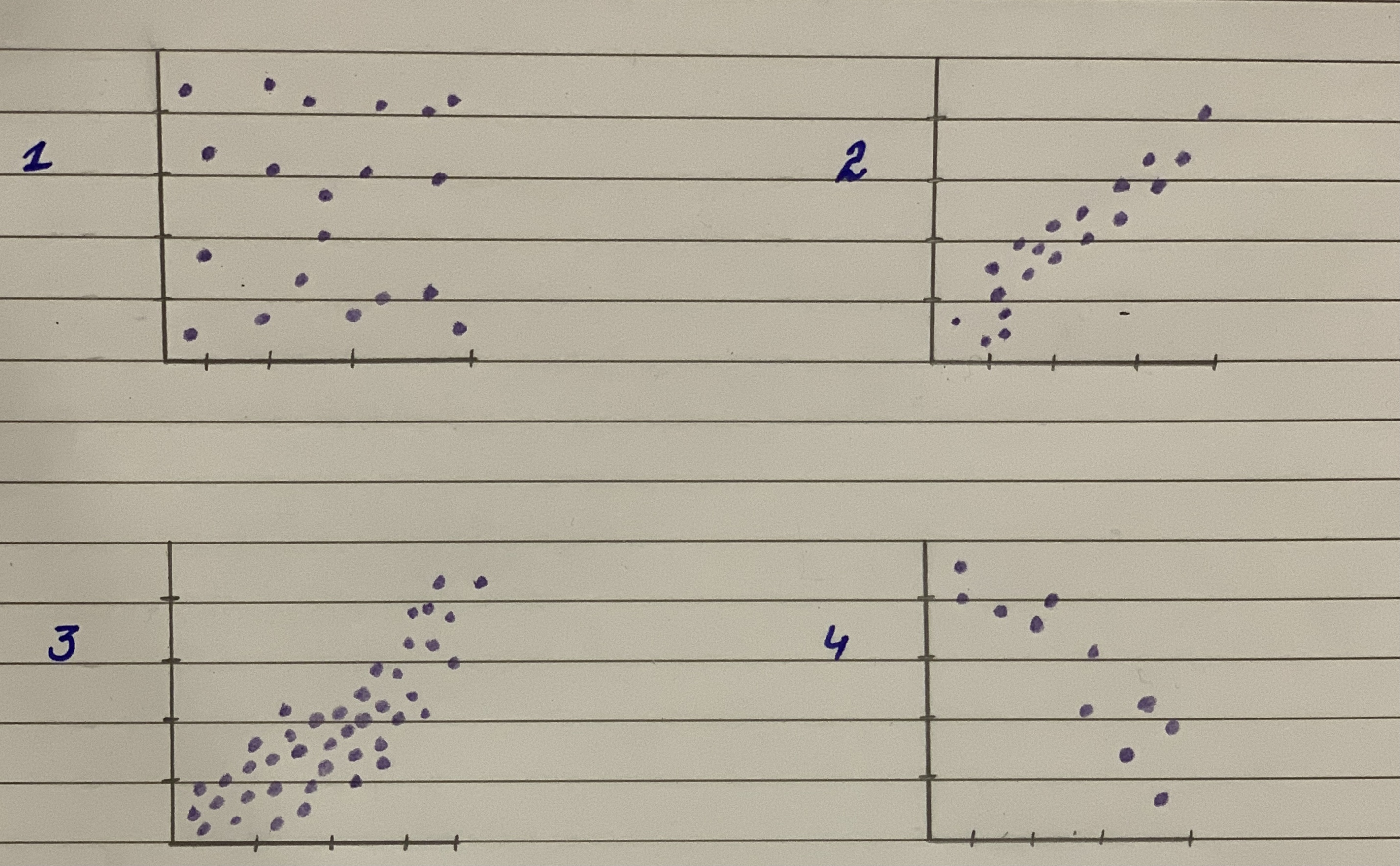Unusual points Each of the four scatterplots that follow shows a cluster of points and one “stray” point. For each, answer these questions:
1) In what way is the point unusual? Does it have high leverage, a large residual, or both?
2) Do you think that point is an influential point?
3) If that point were removed, would the correlation be- come stronger or weaker? Explain.
4) If that point were removed, would the slope of the re- gression line increase or decrease? Explain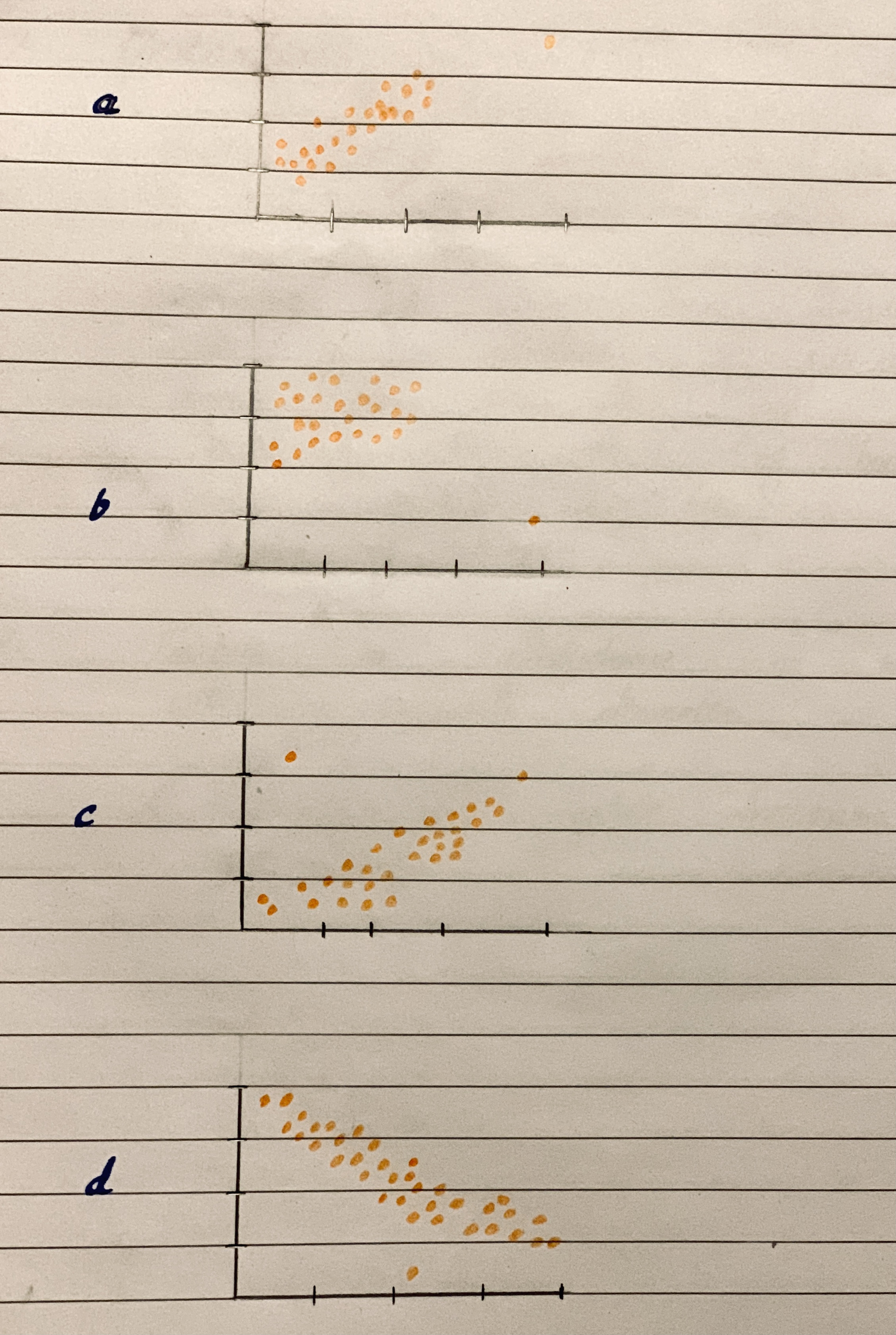Straight Lines Graph the straight lines in Exercises 1–3.
Then find the change in y for a one-unit change in x, find the point at which the line crosses the y-axis, and calculate the value of y when x 52.5.
1. y x 5 1 2.0 0.5
2. y x 5 1 40 36.2
3. y x 5 25 6
Scatterplots For the scatterplots in Exercises 5 describe the pattern that you see. How strong is the pattern? Do you see any outliers or clusters?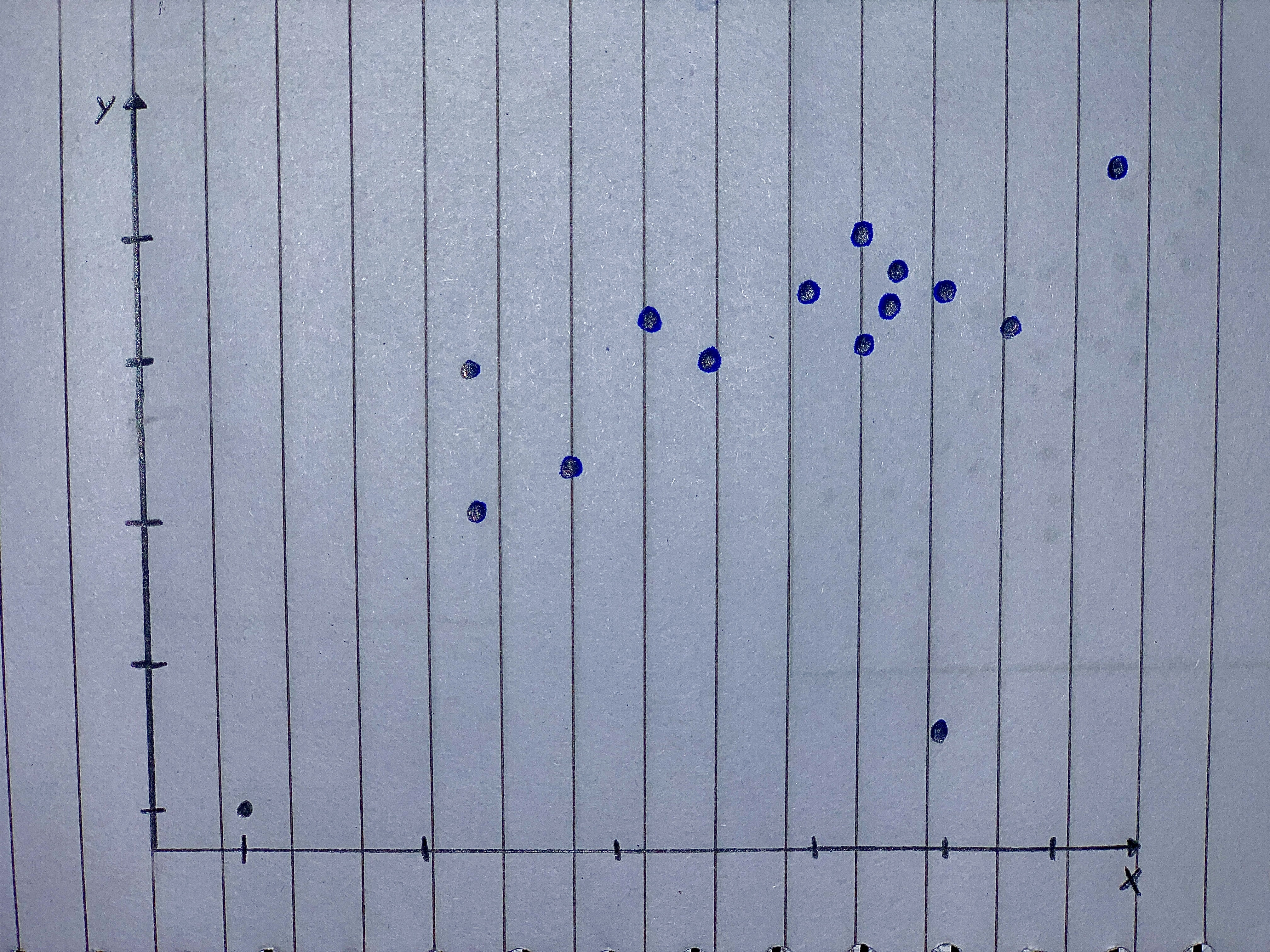Matching Here are several scatterplots. The calculated correlations are -0.923, -0.487, 0.006, and 0.777.
Which is which?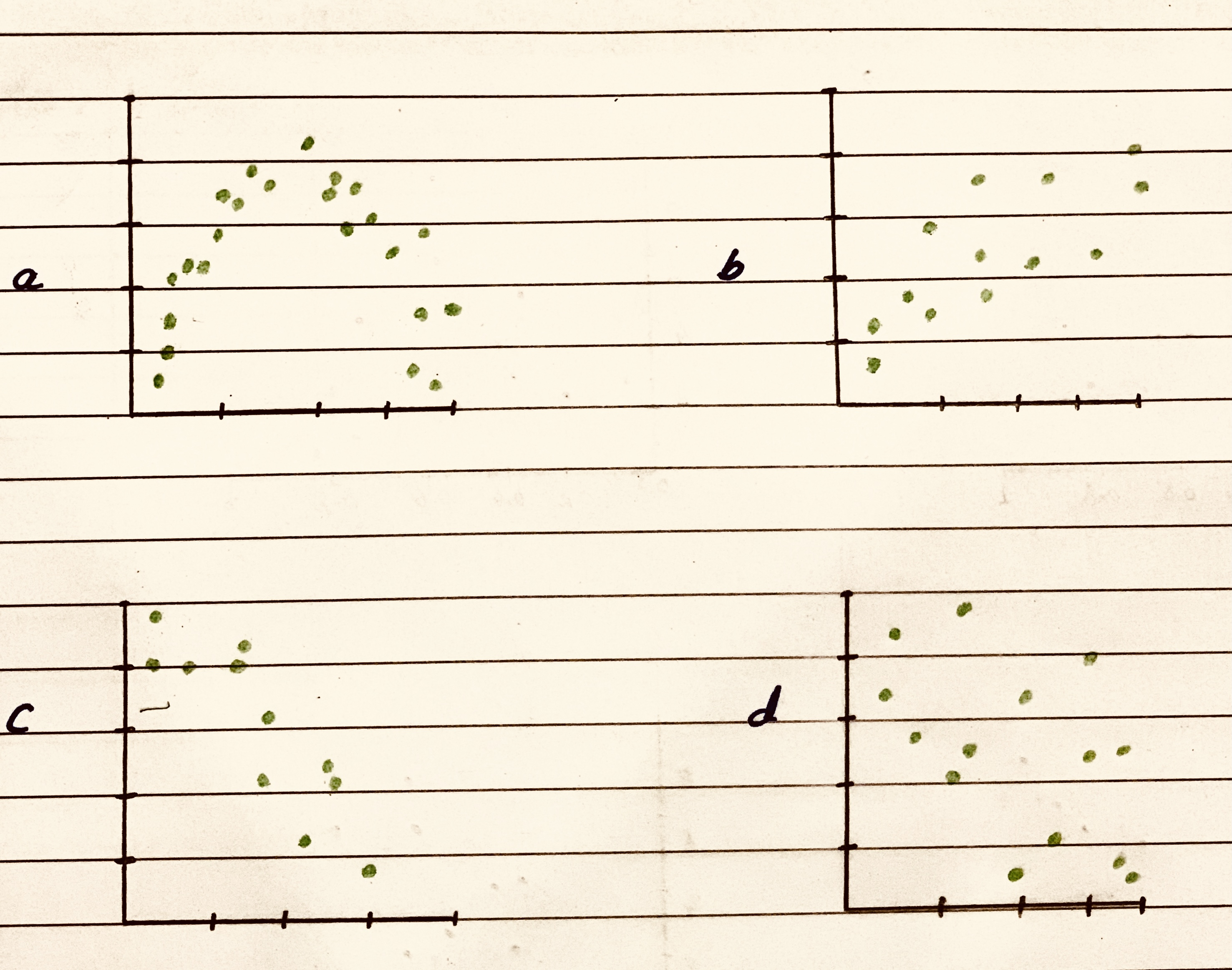Match the values of r with the accompanying scatterplots 0.723,-0.353,0.353,0.998 and -0.998
scatterplots 1,2,3,4,5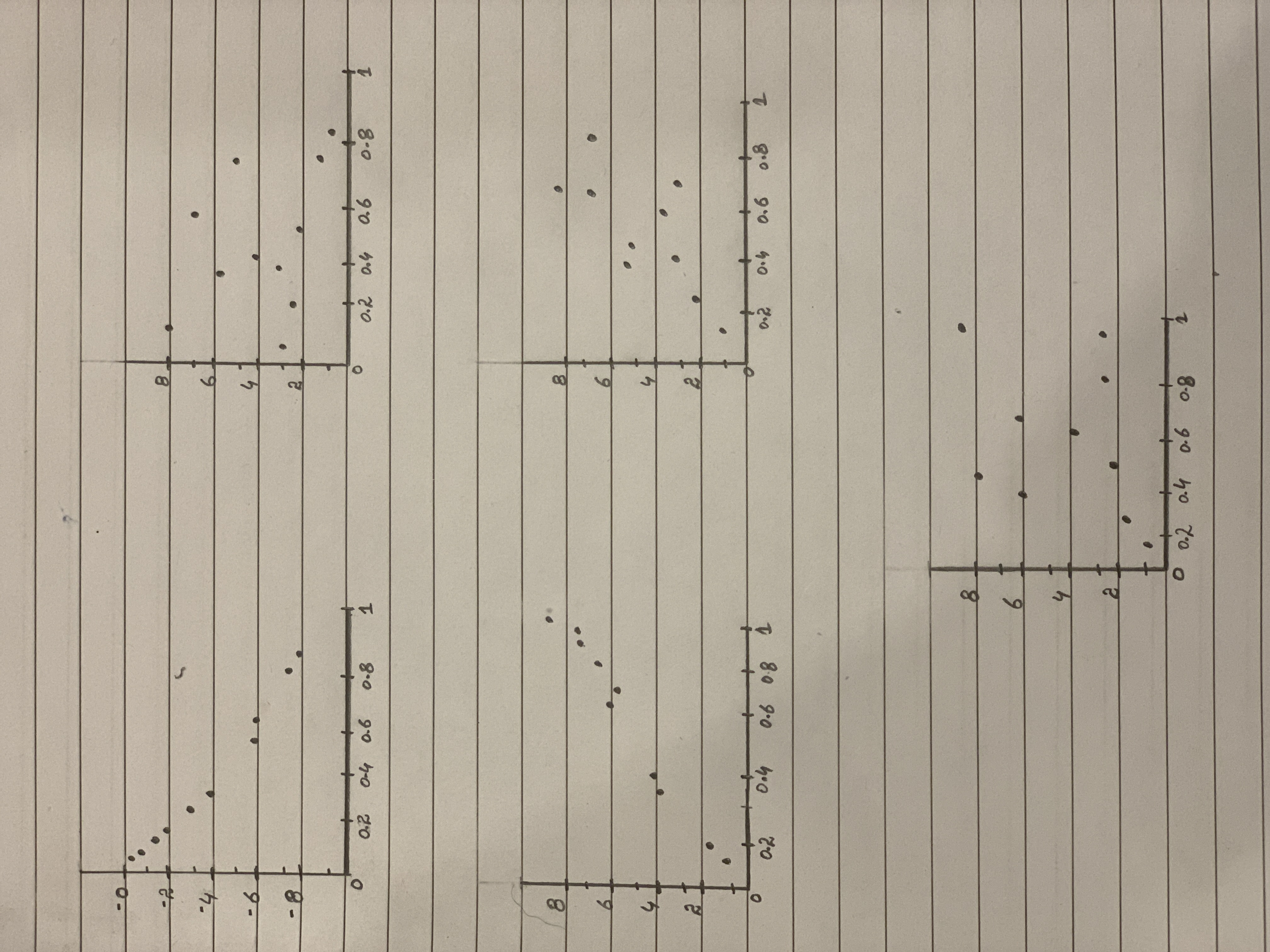The scatterplots below display three bivariate data sets. The correlation coefficients for these data sets are 0.03, 0.68, and 0.89. Which scatter plot corresponds to the data set with r = .03?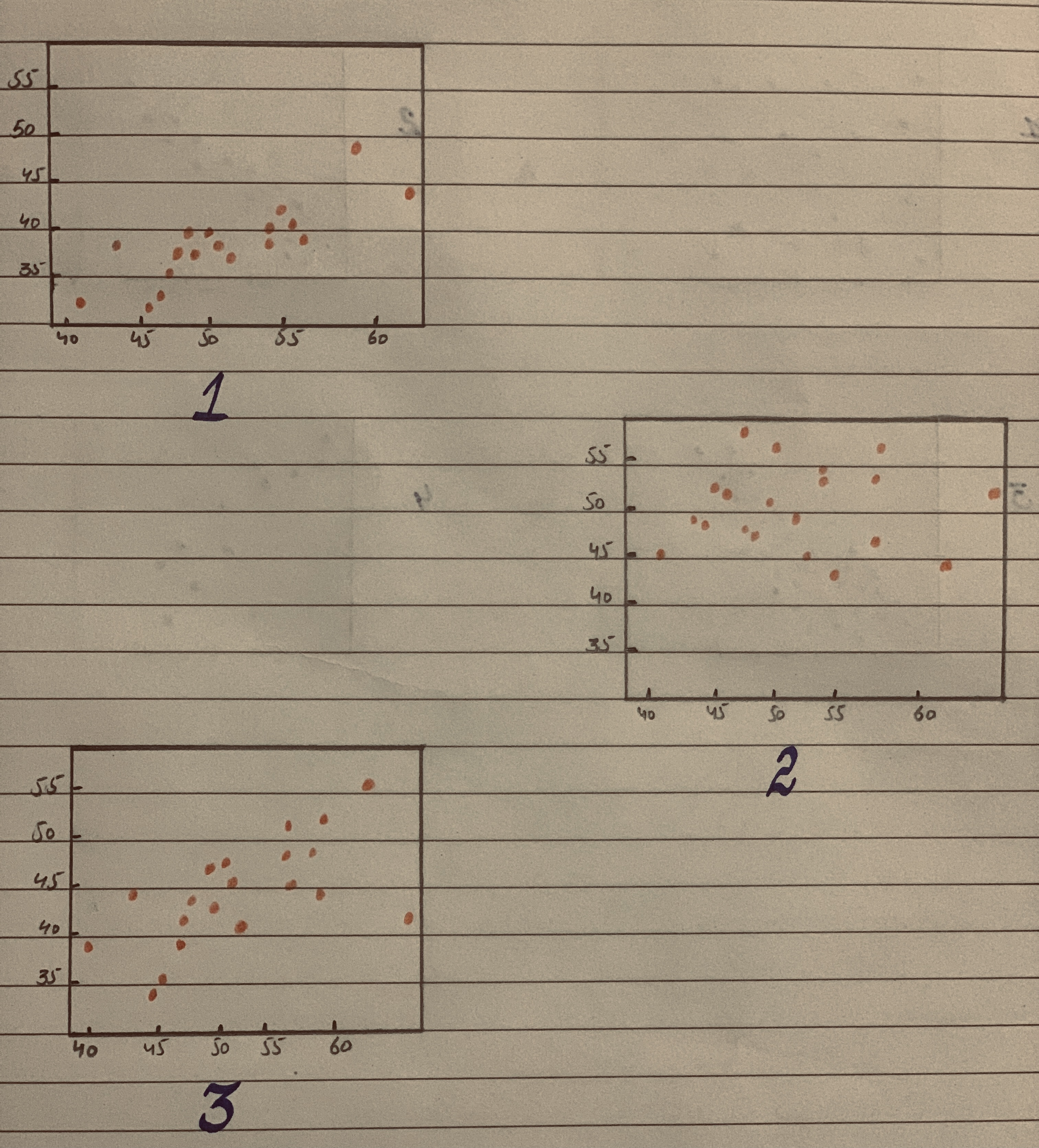Predicting Land Value Both figures concern the assessed value of land (with homes on the land), and both use the same data set. (a). Which do you think has a stronger relationship with value of the land-the number of acres of land or the number of rooms n the homes? Why? b. Il you were trying to predict the value of a parcel of land in e arca (on which there is a home), would you be able to te a better prediction by knowing the acreage or the num- ber of rooms in the house? Explain. (Source: Minitab File, Student 12. "Assess.")